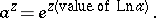# Exponential function

exponent

The functionwhereis the base of the natural logarithm, which is also known as the Napier number. This function is defined for any value of(real or complex) by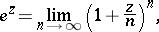(1)

and has the following properties:for any values of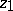and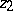.

For real, the graph of(the exponential curve) passes through the point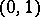and tends asymptotically to the-axis (see Fig.).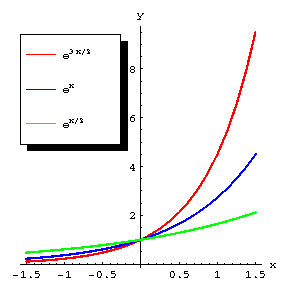Figure: e036910a

In mathematical analysis one considers the exponential function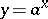for realand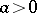,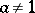; this function is related to the (basic) exponential function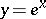by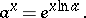The exponential functionis defined for alland is positive, monotone (it increases ifand decreases if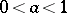), continuous, and infinitely differentiable; moreover,and in particular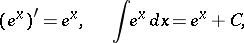and in a neighbourhood of each point the exponential function can be expanded in a power series, for example: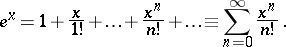(2)

The graph ofis symmetric about the ordinate axis to the graph of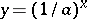. If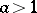,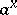increases more rapidly than any power ofas, while asit tends to zero more rapidly than any power of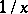, i.e. for any natural number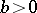,The inverse of an exponential function is a logarithmic function.

Ifandare complex, the exponential function is related to the (basic) exponential functionby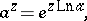whereis the logarithm of the complex number.

The exponential function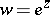is a transcendental function and is the analytic continuation offrom the real axis into the complex plane.

An exponential function can be defined not only by (1) but also by means of the series (2), which converges throughout the complex plane, or by Euler's formula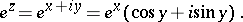If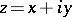, thenThe function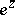is periodic with period: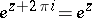. The functionassumes all complex values except zero; the equation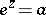has an infinite number of solutions for any complex number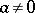. These solutions are given by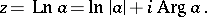The functionis one of the basic elementary functions. It is used to express, for example, the trigonometric and hyperbolic functions.

The basic exponential function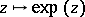defined by (1) or, equivalently, (2) (withinstead of) is single-valued. However, powersforcomplexare multiple-valued sincedenotes the "multiple-valued inverse" to. Thus, since it is customary to abbreviate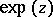as, the left-hand side of the identity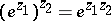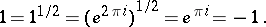By considering a single-valued branch of the logarithm (cf. Branch of an analytic function), or by considering the complete analytic functionon its associated Riemann surface, an awkward notation and a lot of confusion may disappear. For fixed, any value (i.e. determination) of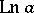defines an exponential function: